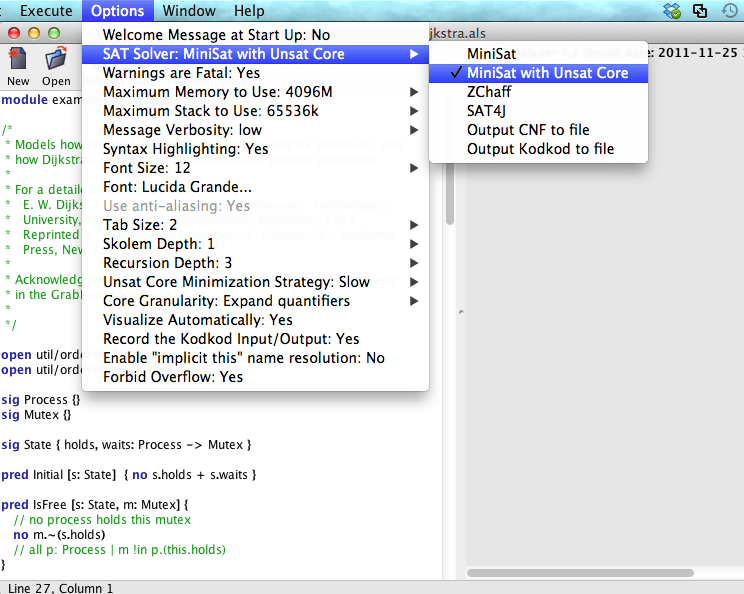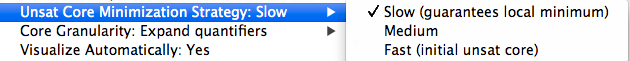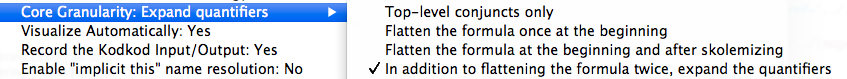An unsatisfiable core is a set of formulas for which no satisfying instance exists. The Alloy Analyzer has a built-in ability to produce an unsat core when it fails to find an instance for a given command. An unsat core can be used to detect potential inconsistencies in a specification (for run command), or check whether an assertion holds vacuously true (for check).

To enable the unsat core generation, select “Minisat with Unsat Core” option for the choice of the SAT solver:When the analyzer fails to find an instance/counterexample for a run/check command, the unsat core can be highlighted by clicking on ‘‘Core’’ in the message panel:Each Alloy constraint that belongs to the unsat core is highlighted red. A constraint highlighted with a bold shade of red is guaranteed to be a member of the core. A constraint highlighted with a lighter shade of red is potentially, but not necessarily, part of the core.

The analyzer allows the user to control two characteristics of an unsat core: core size and granularity.

Core size: The analyzer provides three minimization strategies that determine the size of a resulting core:A core computed using the “Slow” strategy is guaranteed to a local minimum; that is, if any constraint is removed from this core, the resulting set of constraints is satisfiable. The “Fast” strategy takes the least amount of computation, but the resulting core is also the least accurate, and may contain a number of false positives (i.e., constraints that are highlighted light red but not actually part of the core).

Core granularity: By default, each constraint in an unsat core is a top-level conjunct `C<sub>i</sub>` in a given Alloy specification `S` (where `1 <= i <= n`):

`S = C1 /\ C2 /\ ... /\Cn`

However, a top-level conjunct might be too coarse-grained for effectively locating the source of an inconsistency in the specification. For example, consider the following top-level conjunct:

`!(A \/ !B)`

Let us assume that the formula `B` is inconsistent, and the analyze fails to find a satisfying instance for the specification. A resulting unsat core with the default top-level granularity highlights the entire conjunct `!(A \/ !B)`. Now, consider the following equivalent formula, obtained by pushing the negation inside the disjunction:

`!A /\ B`

Given this formula, the analyzer is able to generate a core that highlights only `B`.

The analyzer provides four different types of logical transformations for controlling the granularity of an unsat core (listed in the order of increasing granularity and the amount of required computation):• Top-level conjuncts only: No logical transformation is applied, and constraints in the core correspond to the top-level conjuncts in the given Alloy specification.
• Flatten the formula once at the beginning: The original formulas are transformed into logically equivalent ones by pushing negation in as much as possible (without expanding quantifiers).
• Flatten the formula at the beginning and after skolemnizing: After skolemnization is applied to eliminate existential quantifiers, negation is further pushed into the formulas.
• In addition to flattening the formula twice, expand the quantifiers: Each universal quantifier is fully expanded given the bounds on the signatures, increasing the granularity of a core to parts of the quantified expressions.